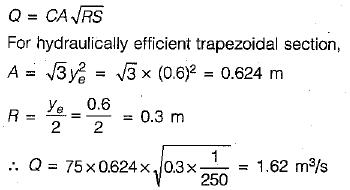Courses

# Flow Through Open Channels - 1

## 10 Questions MCQ Test Mock Test Series for Civil Engineering (CE) GATE 2020 | Flow Through Open Channels - 1

Description
This mock test of Flow Through Open Channels - 1 for Civil Engineering (CE) helps you for every Civil Engineering (CE) entrance exam. This contains 10 Multiple Choice Questions for Civil Engineering (CE) Flow Through Open Channels - 1 (mcq) to study with solutions a complete question bank. The solved questions answers in this Flow Through Open Channels - 1 quiz give you a good mix of easy questions and tough questions. Civil Engineering (CE) students definitely take this Flow Through Open Channels - 1 exercise for a better result in the exam. You can find other Flow Through Open Channels - 1 extra questions, long questions & short questions for Civil Engineering (CE) on EduRev as well by searching above.
QUESTION: 1

### For a wide rectangular channel using Manning’s formula, the differential equation of gradually varied flow (with the usual notations) is given by

Solution: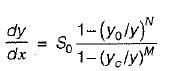For Chezy’s formula N = 3; M = 3
For Manning’s formula N = 10/3; M = 3
N is related to conveyance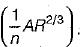QUESTION: 2

### The stage in a river is 4.8 m, the water surface- slope is 1 in 10,000 and the discharge in the stream is 600 m3/s. If the stage remains the same and the water surface slope is 1 in 14,400, then the discharge in the stream will be

Solution: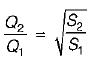∴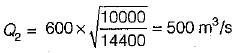QUESTION: 3

### Water surface profiles that are asymptotic at one end and terminated at the other end would include

Solution:

The curves which are asymptotic at one end and terminated at the other end are M2 and H2.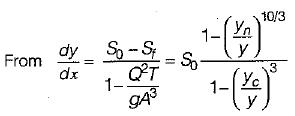Using Manning’s equation for wide rectangular channel.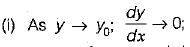i.e. the water surface approaches normal depth line asymptotically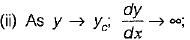i.e. the water surface meets the criticai depth line vertically in region 2 and 3.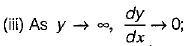i.e. the water surface meets a very large depth as horizontal asymptote in region 1.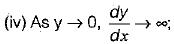it means that the surface profile meets the channel bed vertically in region 3.
Thus,
(i) M1, M2, S2 and S3 meet y0 line asymptotically
(ii) M1 and S1 curve tend to horizontal as y → ∞
(iii) M2, M3 and S2 meet yc line normally
(iv) M3 and S3 meet channel bed. normally.
The slope of:

• C1 and C3 curves comes out to be equal to critical slope of the bed, if Chezy’s equation is used. Otherwise they are slightly curved.
QUESTION: 4

A hydraulic jump is always needed in case of

Solution:

Hydraulic jump is needed when critical depth line is to be crossed.

QUESTION: 5

For a hydraulically efficient rectangular section, the ratio of width to normal depth is

Solution:

For hydraulically efficient rectangular section.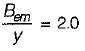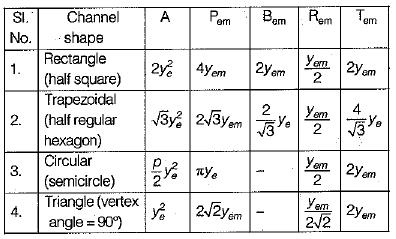QUESTION: 6

Consider the following statements in regard to the critical flow:
1. Specific energy is maximum for a given discharge.
2. Specific force is maximum for a given discharge
3. Discharge is maximum for a given specific force.
4. Discharge is maximum for a given specific energy.

Which of these statements are correct?

Solution:

At critical flow, specific energy and specific force is minimum for a given discharge. In other words for a given specific energy or specific force, discharge is maximum at critical flow.

QUESTION: 7

If F1 and F2 are the Froude numbers of flow before and after the hydraulic jump occurring in a rectangular channel, then

Solution: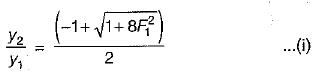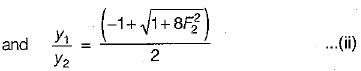Equating the equation (i) with inverse of equation (ii) we get,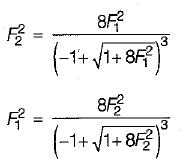QUESTION: 8

The momentum correction factor for a flow through open channel is given by

Solution:
QUESTION: 9

The Froude number of a hydraulic jump is 5.5. The jump can be classified as a/an

Solution:

The hydraulic jumps in horizontal rectangular channels are classified into five categories based on Froude number F1 of the super-critical flow as: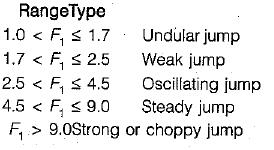QUESTION: 10

A hydraulically efficient trapezoidal section of open channel flow carries water at the optimal depth of 0.6 m. Chezy coefficient is 75 and bed slope is 1 in 250. What is the discharge through the channel?

Solution: